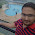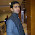How to create pie chart in asp.net using chart control - ASPMANTRA | Asp.Net,MVC,AngularJs,Jquery,Javascript,Sql Server AND WCF snippets and tutorial

# How to create pie chart in asp.net using chart control

In this article I am going to explain how to create pie chart in asp.net using chart control.

Description:
I want to create a pie chart to show the state wise population in percentage. I am using asp.net chart control to create pie chart.

Implementation:
I have created table Tb_Population and insert some dummy records.

Add a webform to project. Drag and drop the chart control from toolbox to webform.

HTML Markup:

<html xmlns="http://www.w3.org/1999/xhtml">
<title></title>
<body>
<form id="form1" runat="server">
<div>
<asp:Chart ID="Chart1" runat="server" Width="800px">
<Legends>
<asp:Legend Alignment="Center" Docking="Left" IsTextAutoFit="true" Name="StateName" LegendStyle="Table"/>
</Legends>
<Series>
<asp:Series Name="Series1" ChartType="Pie"
CustomProperties="PieLineColor=Black, PieLabelStyle=Outside">
</asp:Series>
</Series>
<ChartAreas>
<asp:ChartArea Name="ChartArea1">
<Area3DStyle Enable3D="True" Inclination="0" Rotation="0" />
</asp:ChartArea>
</ChartAreas>
</asp:Chart>
</div>
</form>
</body>
</html>

Import the namespace:

C# Code:
using System.Configuration;
using System.Data.SqlClient;
using System.Data;
using System.Web.UI.DataVisualization.Charting;

VB.net Code:
Imports System.Data
Imports System.Data.SqlClient
Imports System.Configuration
Imports System.Web.UI.DataVisualization.Charting

Create a method to retrieve data from database and show in Chart control.

C# Code
SqlConnection con = new SqlConnection(ConfigurationManager.ConnectionStrings["connection"].ToString());
protected void Page_Load(object sender, EventArgs e)
{
if (!IsPostBack)
{
BindChart();
}
}
public void BindChart()
{
try
{
DataTable dt = new DataTable();
string[] x = new string[dt.Rows.Count];
int[] y = new int[dt.Rows.Count];
for (int i = 0; i < dt.Rows.Count; i++)
{
x[i] = dt.Rows[i].ToString();
y[i] = Convert.ToInt32(dt.Rows[i]);
}
foreach (Series s in Chart1.Series)
{
s.ToolTip = "State: #VALX\nPopulation: #VALY\nPercentage: #PERCENT";
}
Chart1.Series.Points.DataBindXY(x, y);
Chart1.Series.Label = "#PERCENT";
Chart1.Series.LegendText = "#AXISLABEL";
}
catch (Exception ex)
{
}
}

VB.net Code:
Private con As New SqlConnection(ConfigurationManager.ConnectionStrings("connection").ToString())

Protected Sub Page_Load(ByVal sender As Object, ByVal e As System.EventArgs) Handles Me.Load
If Not IsPostBack Then
BindChart()
End If
End Sub
Public Sub BindChart()
Try
Dim dt As New DataTable()
Dim x As String() = New String(dt.Rows.Count - 1) {}
Dim y As Integer() = New Integer(dt.Rows.Count - 1) {}
For i As Integer = 0 To dt.Rows.Count - 1
x(i) = dt.Rows(i)(0).ToString()
y(i) = Convert.ToInt32(dt.Rows(i)(1))
Next
For Each s As Series In Chart1.Series
s.ToolTip = "State: #VALX" & vbLf & "Population: #VALY" & vbLf & "Percentage: #PERCENT"
Next
Chart1.Series(0).Points.DataBindXY(x, y)
Chart1.Series(0).Label = "#PERCENT"
Chart1.Series(0).LegendText = "#AXISLABEL"
Catch ex As Exception
End Try
End Sub

Build the application and run.